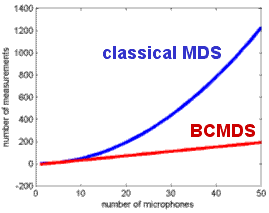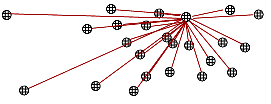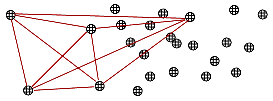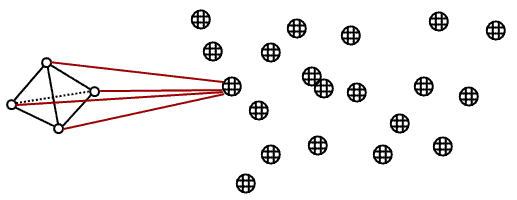# Position Calibration of Microphone Arrays by BCMDS

### Stan Birchfield and Amarnag Subramanya

Applications using microphone arrays, such as acoustic localization, generally require the locations of the microphones to be known.  If these positions are not known when the array is constructed (e.g., a rectangular grid), then the locations must be determined, a problem known as position calibration.  We have developed a rather simple technique for position calibration based upon the idea of multidimensional scaling.

Classical multidimensional scaling (MDS) is an algorithm for determining the Euclidean coordinates of points, given only their interpoint distances.  For example, using the driving distances between cities given in a road atlas, one can construct a map of the region, as shown below.  Although the driving distance is a rough approximation of the actual distance, the resulting map is accurate because the algorithm computes a least-squares solution.Classical MDS requires N2 measurements, where N is the number of points (cities, microphones, etc.) whose coordinates are being estimated.  With a large number of points, it becomes increasingly difficult to obtain the measurements necessary for running classical MDS.  In such a scenario, it is advantageous to take advantage of the redundancy in the squared-distance matrix to reduce the number of measurements needed.  By selecting a small number of the points as basis points, one can  estimate all the interpoint distances from only the distance of each point to the basis points, a tremendous savings in the number of measurements required, which are now linear in the number of points instead of quadratic, as shown below.The technique, which we call Basis-Point Classical MDS (BCMDS), is based on the work of Young and Cliff, 1972.  They showed that, in a P-dimensional space, only P+1 basis points are necessary in order to estimate all the N(N-1)/2 interpoint distances necessary for classical MDS.  Assuming that the basis points are a subset of the microphones, then we need to measure just the P(P+1)/2 distances between the basis points themselves, along with the (P+1)(N-P-1) distances between each non-basis-point and each basis point.  The difference between classical MDS and BCMDS is illustrated below, with the four microphones on the left being used as the basis points.Classical MDS BCMDS

BCMDS is not only useful for large microphone arrays, in which it is impractical to measure all the N2 interpoint distances, but it also enables MDS to be used with a calibration target.  In such a case four speakers are placed on the calibration target, and their relative positions to one another are computed using either classical MDS or some other technique.  Then each of the speakers, in turn, emits a known sound, and the distances between the speaker and all the microphones iare computed by cross-correlating the received signals with the known signal.  The technique requires all the microphones to be synchronized with one another and with the speakers.BCMDS has been shown, through extensive simulations, to be capable of achieving accuracy on the order of 1 cm in everyday indoor environments.  Details of the simulations and the algorithm can be found in the publications below.